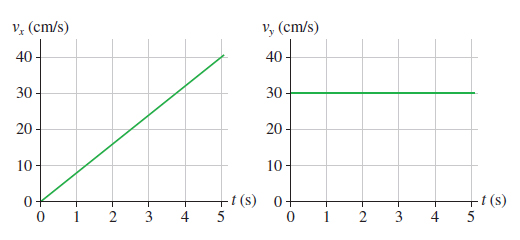# Problem: A rocket-powered hockey puck moves on a horizontal frictionless table. The figure below shows the graphs of vx and vy, the x- and y-components of the puck’s velocity. The puck starts at the origin. You may want to review (Pages 81 - 85).In which direction is the puck moving at exttip{t}{t_1} = 3 s ? Give your answer as an angle from the x-axis.How far from the origin is the puck at 5 s ?

⚠️Our tutors found the solution shown to be helpful for the problem you're searching for. We don't have the exact solution yet.

###### Problem Details
A rocket-powered hockey puck moves on a horizontal frictionless table. The figure below shows the graphs of vx and vy, the x- and y-components of the puck’s velocity. The puck starts at the origin.You may want to review (Pages 81 - 85).

In which direction is the puck moving at = 3 s ? Give your answer as an angle from the x-axis.

How far from the origin is the puck at 5 s ?